# How to Sum Values Based on Month and Year in Excel

We often do some summary or statistic at the end of one month or one year. In these summary tables, there are at least two columns, one column records the date, and the other column records the sales or product number or total costs etc. If we want to calculate the total values based on a certain month of a year, for example if we want to calculate the total sales for January 2020, how can we do? This article will help you to solve this problem.

See the example below. A column lists some dates. B column lists the sales. E column lists the month and year we want to calculate the total sales for. F column is used for showing the returned value by formula.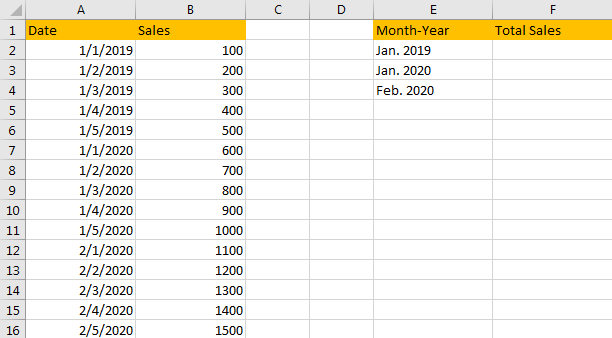## Method 1: Sum Values Based on Month and Year by SUMIF Function

Step 1: In cell F2, enter the formula =SUMIFS(B2:B16,A2:A16,”>=”&DATE(2019,1,1),A2:A16,”<=”&DATE(2019,1,31)).

In SUMIFS function, B2:B16 is the sum range, A2:A16 is the criteria range. “>=”&DATE(2019,1,1) and “<=”&DATE(2019,1,31) are the two criteria. Details please see below screenshot.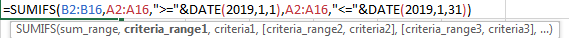Verify that total sales 1500 is displayed for January 2019 after calculation.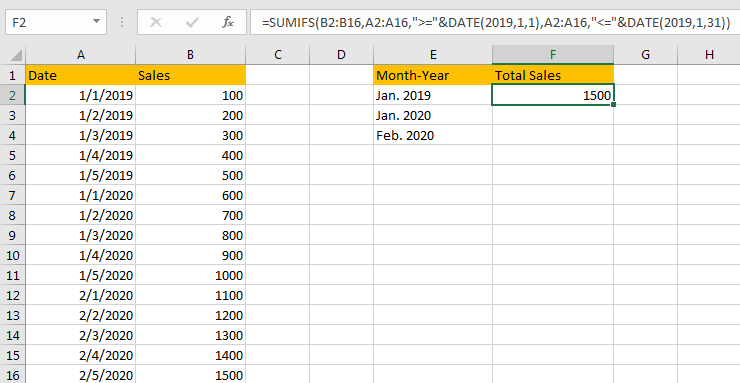Step 2: If we want to calculate total sales based on a certain period, we can change the parameters in DATE function. For example, to calculate the total sales for period 1/3/2019 – 1/3/2020, we can enter the formula =SUMIFS(B2:B16,A2:A16,”>=”&DATE(2019,1,3),A2:A16,”<=”&DATE(2020,1,3)).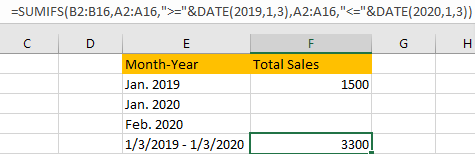Above all, you can change the date in DATE function to do sum per your demands.

## Method 2: Sum Values Based on Month and Year by SUMPRODUCT

Step 1: In cell F2, enter the formula

=SUMPRODUCT((MONTH(A2:A16)=1)*(12(A2:A16)=2019)*(B2:B16)).

In this formula we also use MONTH function and YEAR function to filter date from range A2:A16 based on criteria ‘Date is included in period January 2019’. B2:B16 is the sum range.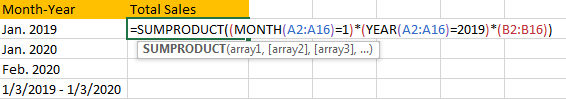Step 2: Click Enter to get the result. Verify that we get the same result in method #1 step#1.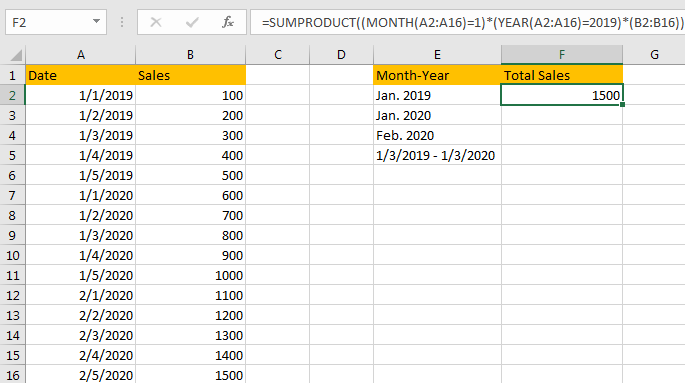### Related Functions

• Excel SUMPRODUCT function
The Excel SUMPRODUCT function multiplies corresponding components in the given one or more arrays or ranges, and returns the sum of those products.The syntax of the SUMPRODUCT function is as below:= SUMPRODUCT (array1,[array2],…)…
• Excel MONTH Function
The Excel MONTH function returns the month of a date represented by a serial number. And the month is an integer number from 1 to 12.The syntax of the MONTH function is as below:=MONTH (serial_number)…
• Excel DATE function
The Excel DATE function returns the serial number for a date.The syntax of the DATE function is as below:= DATE (year, month, day)…
• Excel YEAR function
The Excel YEAR function returns a four-digit year from a given date value, the year is returned as an integer ranging from 1900 to 9999. The syntax of the YEAR function is as below:=YEAR (serial_number)…

Related Posts

How to Count Dates of Given Year in Excel

This post will guide you how to count Dates of a certain year in the range of dates using a formula in Excel 2013/2016 or Excel office 365. How do I count dates by a given year in Excel. And ...

How to Sum Every Nth Column in Excel

Sometimes for data listed in rows, we may want to sum data every Nth column, for example sum data only in ODD column or EVEN column (every 2 column). In our daily life, we may meet many cases like this. ...

How to Count Dates by Day of Week in Excel

This post will guide you how to count days of week in the range of dates using a formula in Excel 2013/2016 or Excel office 365. How do I count dates by day of week in Excel. Count Dates by ...

How to Count Cells that do not Contain Errors in Excel

This post will guide you how to count the number of cells that do not contain errors within a range of cells using a formula in Excel 2013/2016 or Excel office 365. How do I count the number of cells ...

How to Convert Date & Time Format to Date in Excel

Sometimes we want to convert date and time format to date only format in excel for example convert 01/29/2019 06:51:03 to 01/29/2019, we can convert format by Formula or Format Settings. The two ways are easy to learn, so you ...

How to Extract Year from Date & Time Format in Excel

Sometimes we want to get Year information from date and time format to show the Year only in excel. For example convert 01/29/2019 06:51:03 to 2019, we can get Year information by Formula or Format Settings. The two ways are ...

How to Calculate Remaining Days in a Month or Year in Excel

This post will guide you how to calculate remaining days in a given month or year in Excel. How do I calculate the number of days left in a month or year using a formula in Excel 2013/2016. Calculate Remaining ...

How to Split Date into Day, Month and Year in Excel

This post will guide you how to split date into separate day, month and year in excel. How do I quickly split date as Day, Month and Year using Formulas or Text to Columns feature in Excel. Split Date into ...

How to sort Dates by Month and Day Only in Excel

This post will guide you how to sort dates or birthdays by month or day only in Excel. How do I sort dates by Year or Month or Day only with a formula in Excel 2013/2016. How to use the ...

How to Add the Current Month or Year in a Cell in Excel

This post will guide you how to add the current month or year into a cell or header or footer in your worksheet. How do I insert the current date or time in a cell with a formula in Excel. ...

Sidebar